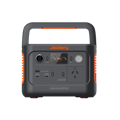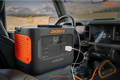## Ultimate Guide to Milliamps to Amps (mA to A) Conversion

Milliamps (mA) and amperes (A) are both electrical current units, but they are different magnitudes. mA is a smaller unit than A. Ampere, generally abbreviated as amp, is the basic or SI unit of the electric current. In contrast, a milliampere (or mA) is 1/1000 of an ampere (or A).

In mathematical terms, 1 amp is equal to 1000 milliamps. You can use this equation to convert mA to A or vice versa. If you are planning to invest in solar power stations, you'll need to look for their amps or milliamps value and match it with the power requirements of your appliances.

Generally, the higher the value of amps, the better your solar power station. Jackery Explorer Portable Power Stations come with high wattage and ampere value. Jackery Explorer 300 Plus Power Station is a compact solar charging solution with a battery capacity of 293Wh or 20.4Ah. It weighs only 7.1 lbs, making it suitable for outdoor adventures.

- Portable: 12.8V/22.5Ah/3.75Kg, fits in your backpack

- Long-lasting: Features an LFP battery with a 10-year lifespan.

- Ultra-Safe: High FCC/CE and UL certifications, innovative ChargeShield Technology (52 forms of protection).

- Sustainable: Whisper-quiet, emission-free, and offers 4-way charging (solar, mains, car lighter, type-C).## What is Milliamps to Amps (mA to A)

Milliampere is the multiple of ampere, an SI unit of electric current. The prefix "milli" generally means one-thousandths or . The small amounts of electrical current flowing through the conductor are typically measured by milliamperes.

In other words, a milliamp specifies the battery capacity. One example of a milliamp is a mobile phone battery specified by 3000 milliamp-hour. This means the battery can supply around 3000 mA for 1 hour.

The amperage, also called amp, is the electric current constant equivalent to the flow of one coulomb per second. In the metric system, the SI unit for electric current is amp.

Ampere is also used to measure the electric battery's capacity. Common home appliances like kettles, electric fires, refrigerators, etc., are measured using amperes.
The milliamp (mA) is equal to 1/1000 of an ampere (A) and is abbreviated as 1 A = 1000 mA or 1 mA = 0.001 A.
Amperage and Ohm's Law
According to Ohm's law, the current between two points in a conductor is proportional to voltage and inversely proportional to resistance.
Here is how Ohm's law is expressed:
I = V / R
In other words, the current (abbreviated in amps) is equal to the voltage (abbreviated in volts) and resistance (abbreviated in ohms).

## How to Convert mA to A

If you want to convert mA to A, all you need to do is divide the value of milliamperes by 1000.
Formula: A = mA ÷ 1000.
Here are some examples of converting milliamps to amps (mA to A).
Example 1: Convert 3000mA to Ampere (A)
Amps (A) = 3000mA / 1000 = 3A
Example 2: Convert 1500mA to Ampere (A)
Amps (A) = 1500mA / 1000 = 1.5A
Example 3: The most popular Jackery Explorer Power Stations has a high milliamp rating of 50,000 mAh. You can convert milliamp-hour to amp-hour using the below formula.
Amps-hour = 50,000mAh / 1000 = 50Ah
Milliamps represent small electric currents, especially in devices like laptops, smartphones, etc. While amps are used in larger electrical appliances like refrigerators, air conditioners, etc.
Converting mA to A lets you work with electric currents more practically and ensures consistency when comparing appliances with different electric units.
Below are some of the typical mA to A conversions.

Milliampere [mA]

Ampere [A]

0.01 mA

1.0E-5 A

0.1 mA

0.0001 A

1 mA

0.001 A

2 mA

0.002 A

3 mA

0.003 A

5 mA

0.005 A

10 mA

0.01 A

20 mA

0.02 A

50 mA

0.05 A

100 mA

0.1 A

## How to Convert A to mA

Similar to mA to A conversion, you can convert amps to milliamps (A to mA) using one formula.
Formula: mA = A × 1000

Example 1: Convert 5A to mA

mA = 5A × 1000 = 5000mA

Example 2: Convert 10A to mA

mA = 10A × 1000 = 10,000mA
Example 3: Convert 1.5A to mA
mA = 1.5A × 1000 = 1500mA
While amperes are common in power systems, there are instances when you'll need to convert amps to milliamps. It is of paramount importance when you are dealing with multiple values. Converting the mixture of small and large currents ensures consistency and simplifies comparison.
Below are some common A to mA conversions.

Amps (A)

Milliamps (mA)

0.01 A

10 mA

0.05 A

50 mA

1 A

1000 mA

2 A

2000 mA

3 A

3000 mA

4 A

4000 mA

5 A

5000 mA

## How to Convert mA to All

Converting milliamperes (mA) to amperes (A) and more is a fundamental skill that allows for seamless transitions between units of electric current. In this section, we will learn how to convert mA to A, as well as other units of current, such as microamps (μA), nanoamps (nA), and kiloamperes (kA).
Abamperes (abA): Abamperes, also called biot (Bi), is the unit of electric current in the cgs-emu (centimeter-gram-second electromagnetic) system of units. It is generally abbreviated as abA.
Gigaamperes (GA): It is the SI-multiple of the electric current unit ampere and is generally equal to 1 billion amperes.
Gilberts (Gb): An obsolete unit of the electric current generally used in practical cgs and CGS-EMU systems for measuring magnetomotive force.
Kiloamperes (kA): It is the multiple of ampere, which is the SI unit for electric current. One kiloampere of electric current is equal to 1000 amperes.

Megaamperes (MA): One megaampere of electric current is equivalent to 1,000,000 ampere. In the current metric system, the prefix "mega" is for millions or.

Microamperes (μA): It is the unit of electric current, which is equal to one-millionth of an ampere.
Nanoamperes (nA): The SI unit of electrical current whose value is equal to of an ampere.
Ah, or amp-hour, is one of the most important terms that describe battery capacity. For instance, a fully charged 20Ah power station is capable of providing 20 amps of current for one hour or 5 amps of current for 4 hours.
In the below table, we will convert 1 mA to some common electric-current units like amperes, abamperes, gigaamperes, kiloamperes, microamperes, etc.

1 Milliampere

to common electric-current units

1 mA

= 0.001 amperes (A)

1 mA

= 0.0001 abamperes (abA)

1 mA

= 1.0E-12 gigaamperes (GA)

1 mA

= 0.0012566366121077 gilberts (Gb)

1 mA

= 0.0001 biots (Bi)

1 mA

= 1.0E-6 kiloamperes (kA)

1 mA

= 1.0E-9 megaamperes (MA)

1 mA

= 1000 microamperes (μA)

1 mA

= 1 milliamperes (mA)

1 mA

= 1000000 nanoamperes (nA)

## Jackery Power Stations Explained

Amp-hours is the unit that measures the capacity of a power station or battery. The Ah rating defines the amount of current a battery can provide at a specific rate for a particular time. When selecting the right power station, you'll need to note its amp hours.
Jackery is a renowned solar brand manufacturer of high-quality solar panels, solar generators, and power stations. They generally have a higher watt-hour and amp-hour value. This means these power stations can supply electricity to appliances for long hours.

### Jackery Explorer 300 Plus

The Jackery 300 Plus has a capacity of 288Wh and an output of 300W power, with dual PD ports capable of up to 100W output, supporting fast charging for multiple devices simultaneously, and offering various charging options, making it convenient for outdoor charging.Jackery Explorer 300 Plus

### Jackery Explorer 500

Jackery Explorer 500 Portable Power Station has a battery capacity of 518Wh. It weighs only 13.32 lbs and has a foldable handle with an ergonomic design for portability. The compact and lightweight power station can charge low to high-power appliances, including TV, mini-refrigerators, CPAP machines, air pumps, and more.Jackery Explorer 500

### Jackery Explorer 1000 Pro

Equipped with a battery capacity of 1002Wh, the Jackery Explorer 1000 Pro Portable Power Station is ideal for powering appliances during power outages and camping. It features multiple output ports to charge eight devices simultaneously. You can recharge the power station using wall charging, car outlet, and solar charging.Jackery Explorer 1000 Pro

Below we will reveal how long Jackery power stations can charge your home or outdoor appliances.

Series

Appliances

Camera (8.4W): 13 Charges

Gaming Console (18W): 10 Times

Phone (29W): 13 Times

CPAP (20W): 11 H

Refrigerator (90W): 2.5 H

Drone (90W): 5 Times

Phone (18W): 22.6 Charges

TV (60W): 7.5 H

Blender (300W): 7 H

Space Heater (350W): 1.5 H

Coffee Maker (550W): 1.5 H

Toaster (650W): 1.3 H

Microwave (700W): 1.2 H

Kettle (850W): 1 H

Ice Shaver (700W): 1.2 H

Space Heater (350W): 2.5 H

Blender (300W): 2.5 H

## Milliamps to Amps (mA to A) FAQs

1. How many mA is equal to 1A?

One milliampere is equal to 1/1000 of an ampere. In other words, the milliampere is the multiple of an ampere, which is the electrical current equivalent to the flow of one coulomb per second. You can easily convert mA to A using the formula: mA = 1/1000A.

2. How to convert mAh to Wh?

Watt-hour, abbreviated as Wh, is the energy unit equal to one watt of power generated or consumed in one hour. Watt-hour and milliamp-hour both are generally used to describe the battery capacity.

The simple formula to convert mAh to Wh is:

Watt-hours (Wh) = milliampere (mAh) × Volts (V) ÷ 1000

For instance, if the power station has a mAh of 50,000 and 120V, the battery capacity in watt-hour would be:

Wh = 50,000mAh × 120V ÷ 1000 = 6000Wh.

Below we will convert some common mAh values to Wh.

Milliamp Hours (mAh)

Voltage (V)

Watt Hours (Wh)

1mAh

3.7V

0.0037Wh

1,000mAh

3.7V

3.7Wh

1,200mAh

3.7V

4.44Wh

2,000mAh

3.7V

7.4Wh

2,500mAh

3.7V

9.25Wh

3,000mAh

3.7V

11.1Wh

4,000mAh

3.7V

14.8Wh

4,500mAh

3.7V

16.65Wh

5,000mAh

3.7V

18.5Wh

3. What are the relations of amps, watts, and volts?

Amps, watts, and volts are necessary critical units you'll come across when dealing with electricity or power stations.

Amps: Amperage measures the number of electrons that flow through a certain point per second. The larger the amps, the more electricity can flow through the circuit.

Watts: Wattage is the energy that a particular electric appliance consumes. The higher the watts, the more power the device consumes.
Volts: It measures the force or pressure the electric current requires to pass through the electric wire.
Volts, watts, and amps are related to one another as follows:
Watts = Amps × Volts

## Final Thoughts

Converting mA to A becomes necessary when it comes to purchasing a solar power bank or generator. You can compare the mAh value of your appliances and find a compatible battery system to provide power.

Jackery Explorer Power Stations and Solar Generators are reliable power sources capable of charging most small and large appliances.

The finest range of Jackery power stations includes portable batteries with capacities ranging from 300Wh to 3000Wh and more. Depending on your power needs, consider choosing the right-fit power station to charge most of your home or outdoor appliances.

### Best-selling Jackery Portable Power Stations

Sale Off### Jackery Explorer 2000 Plus Portable Power Station

\$3,599.00\$3,419.00
Sale Off### Jackery Explorer 2000 Pro Portable Power Station

\$3,299.00
Sale Off### Jackery Explorer 1000 Pro Portable Power Station

\$1,999.00\$1,699.00

### New Arrival

Hurry up! Sale ends once the timer hits zero

00Days
00Hrs
00Mins
00Secs